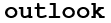﻿ One Mathematical Cat, Please! Ideas for Anyone Who Wants to Understand Mathematics

# One Mathematical Cat, Please! Ideas for Anyone Who Wants to Understand Mathematics

Want a fresh start on math? View it in a totally new way? Then read this little book.

I call it the ‘cat-on-swing’ book because of the print-version cover.

It can change your relationship with math in 142 easy pages. Read it for free below, or get a print copy at Amazon.

Note: If you want my entire Algebra course, then check out my cat-in-hammock book. The cat-on-swing book (discussed on this page) is embedded in the cat-in-hammock book, so you don't need both!

If you're using my higher-level materials (Geometry, Algebra II, Precalculus, Calculus) without having gone through my Algebra I course, then I highly recommend that you read the cat-on-swing book. It will quickly bring you up-to-speed with all the math language ideas you need to fully benefit from my lessons.

You'll learn that numbers have lots of different names, and math is the renaming tool. You'll learn that ‘$\,x\,$’ is to math as ‘cat’ is to English. It's an easy read. If you can add, subtract, multiply and divide with numbers like 1, 2, and 3, then you can read this book.

Supplement any math class, 7th grade through college. Use for self-learners, home-schoolers, math lovers, math haters. Reviewers and users write:

• “... wonderfully written and crafted with a care you rarely see”
• “... will do a great service to the mathematical educational world”
• “... the need for this book is immense”
• “... I found meat, potatoes, and pie on every page. It's all dessert.”
• “... never seen anything so supportive and affirming and reassuring and inspiring as the way you talk us through topics”
• “THIS IS GREAT!!!! . . . this is helping me get heads above the competition. You rock!!!! One Mathematical Cat drives me wild.”

An entire website grew from this book. Free randomly-generated exercises. Free unlimited worksheets. Algebra Pinball. All the same style. All the same voice. All building on the ideas in this little book.

If you have any questions, don't hesitate to contact me (Dr. Carol JVF Burns, author and website creator):(the at sign)(dot)This email is a mix of graphics and text to try and foil spammers (sigh).

## Read the Original Cat Book(Cat-On-Swing)

GREEN ENTRIES are web exercises that support the book. Be sure to visit these pages and click-click-click after reading each section!
English versus Mathematics
This diagram summarizes the language ideas discussed in the first section, The Language of Mathematics.
1.  THE LANGUAGE OF MATHEMATICS (pages 1–12)
expressions versus sentences; sentences have verbs and state a complete thought; conventions; definitions; simplifying an expression; connectives
2.  THE REAL NUMBERS (pages 13–25)
the real numbers and where they live; living to the right or left of zero; size (distance from zero) versus order (left/right positioning); opposites; integers; averaging
3.  MATHEMATICIANS ARE FOND OF COLLECTIONS (pages 26–39)
introduction to sets; membership in a set; finite versus infinite sets; notation used in connection with sets; the idea of ‘infinity’; interval notation
introduction to sets
4.  HOLDING THIS, HOLDING THAT (pages 40–57)
letters used to represent other objects—variables; variables ‘hold’ objects; variables are used to state a general principle, represent a sequence of operations, represent an ‘unknown’ quantity; solving a sentence
introduction to variables
going from a sequence of operations to an expression
going from an expression to a sequence of operations
solving simple sentences by inspection
using mathematical conventions
5.  I LIVE TWO BLOCKS WEST OF YOU (pages 58–74)
order properties of the real numbers—position of one number relative to another; mathematical sentences used to describe order relationships; the phrases ‘greatest’, ‘least’, ‘at least’, ‘at most’; describing a whole class of sentences in one fell swoop; equations versus inequalities; solution of a sentence in one variable; graph of a sentence; reading a sentence backwards
identifying inequalities as true or false
solving equations of the form $\,xy = 0\,$
6.  NUMBERS HAVE LOTS OF DIFFERENT NAMES! (pages 75–93)
different names can reveal various properties that a number has; theorems are to a mathematician as tools are to a carpenter; the power of adding zero and multiplying by one; simplifying an expression versus solving a sentence; unit conversion
7.  THESE SENTENCES CERTAINLY LOOK DIFFERENT (pages 94–111)
comparing expressions versus comparing sentences; equality of expressions versus equivalence of sentences; connectives; truth tables; synonyms for ‘is equivalent to’; the mathematical words ‘and’ and ‘or’
8.  TRANSFORMING TOOL #1 (the Addition Property of Equality) (pages 112–127)
the Addition Property of Equality; facts versus commands; ‘for all’ sentences; translating a theorem; preferred formats for solving a sentence; why the Addition Property of Equality is true; preferred format for checking solutions
9.  TRANSFORMING TOOL #2 (the Multiplication Property of Equality) (pages 128–142)
the Multiplication Property of Equality; what goes wrong with multiplying by zero; undoing the last operation first; why the Multiplication Property of Equality is true
SAMPLE QUIZZES:
Students at Miss Hall's School were given the following quizzes, to test their understanding of each chapter.